## Wednesday, December 27, 2017

Unit – 2
Theory of Consumer Behaviour
A. Very Short Type Questions (Mark – 1):
1. What is meant by utility? 2014
Ans. Utility refers to the want satisfying capacity of a commodity.
2. Define total utility?
Ans. Total utility is the sum of utility derived from the consumption of all units of a commodity.
3. Define marginal utility?
Ans. Marginal utility refers to additional utility due to consumption of an additional unit of commodity.
4. What is utility function? 2013
Ans: In economics, the utility function measures welfare or satisfaction of a consumer as a function of consumption of real goods, such as food, clothing and composite goods rather than nominal goods measured in nominal terms. Utility function is widely used in the rational choice theory to analyze human behavior.
5. Explain the relationship between total utility and marginal utility?
Ans: Relationship between Total Utility and Marginal Utility are as follows – (a) TU = MU, (b) TU increases as long as MU is positive, (c) When MU is zero, TU is maximum, (d) When MU is negative, TU starts diminishing, (e) Decreasing MU implies that TU is increasing at decreasing rate.
6. What is law of diminishing marginal utility?

Ans. Law of diminishing marginal utility states that as the consumer consumes more and more units of the commodity, marginal utility goes on falling.
7. What is law of equimarginal utility?
Ans. Law of equi-marginal utility states that a consumer gets maximum satisfaction when the ratio of the marginal utilities of two goods and their prices are equal
8. Define price line.
Ans. Price line is a line showing different combination of two goods which consumer can attain, given income and market price of the goods. In other words, Graphical representation of budget set.
9. Define budget set?
Ans. Budget set refers to a set of attainable combination of two goods, given market price of the goods and income of the consumer.
10. Define budget line? Define the slope of budget line. 2013
Ans. Budget line is a straight line curve which indicates different combination of commodities that the consumer can purchased with his money income.
Slope of budget line represents the ratio of the prices of two goods in such a manner that both goods are equal to the consumer. That means, slope of budget line is equal to the ratio of the prices of the two commodities i.e.  - PX/PY. Its slopes downwards because the consumer can buy extra units of one commodity only by sacrificing some units of the other commodity.
Another characteristic of budget is that it is a straight line implying that slope of budget line is constant. The slope is constant because price ratio remains constant.
11. What does the slope of the consumer’s budget line indicate?
Ans. Slope of consumer budget line indicates that the consumer is willing to for go for an additional unit of another good. That means slope of budget line indicates the marginal rate of substitution of two goods.
12. What do the points below the consumer budget line indicate?
Ans. The lower points of budget line indicate the lower amount of goods than the points on the budget line.
13. What does the rate of substitution between two goods measure?
Ans. MRS of X for Y refers the consumer is willing to for go for an additional unit of goods X for Y.
14. What is an indifference curve?
Ans. An indifference curve shows the various combination of two goods, which give the same total satisfaction to the consumer.
15. What id meant by indifference set?
Ans. Indifference set refers to those combinations of two goods which offer the consumer the same level of satisfaction.
16. Define indifference map?
Ans. A set of indifference curves placed together in a diagram is known as indifference map.
17. Define marginal rate of substitution? 2016
Ans. The marginal rate of substitution refers to the rate at which the consumer is willing to sacrifice goods – Y for a unit more of goods – X.
18. What does an indifference curve slope downwards? 2013
Ans: The indifference curve slope downwards from left to right because the consumer increases more units of one commodity he will have to sacrifice the another commodity so that level of satisfaction is maintained.
19. What do you mean by monotonic preferences?
Ans. Monotonic preferences imply that any point above the indifference curve represents a bundle which is preferred to the bundles on the indifference curve.
20. What is indifference map?
Ans. Indifference map represents a collection of indifference curves where each curve represents a certain level of satisfaction of the consumer.
21. What is consumer’s equilibrium?
Ans. The point at which the budget line is tangent to an indifference curve will be the optimum choice of the consumer which will give him the maximum possible satisfaction. This is called consumer’s equilibrium.
22. The slope of the demand curve measures the ____ at which demand changes with respect to its price.
Ans. Rate of change (Elasticity)
23. What is demand function?
Ans. The demand function for a commodity is the relation between the various quantities of the commodity. If the price of x commodity increase then there is change in demand for x commodity.
24. What do you mean by demand?
Ans. By demand means the various quantities of a given commodity which an individual is willing to purchase at different prices within a given period of time.
25. Mention two determinant of demand?
Ans. (i) Price of the commodity; (ii) Income of the individual consumer.
26. Write the law of demand?
Ans. The law of demand states that “Other things remains constant” when the price of the commodity falls, demand increases and vice-versa. That means there is an inverse relationship between price of the commodity and its quantity.
27. Give two example of joint demand.
Ans. (i) Pen and Ink; (ii) Tea, Milk and sugar
28. What is demand curve?
Ans. Demand curve is a graphic presentation of demand schedule showing inverse relationship between price and quantity demanded of a commodity.
29. What is the slope of demand curve like?
Ans. Demand curve slopes downward from left to right, indicating inverse relationship between price and quantity demanded of a commodity.
30. Define Giffen goods.
Ans. Giffen goods are those inferior goods in the case of which there is a positive relationship between price and quantity demanded. A good is called a Giffen good as it satisfies the following three conditions.
1. It should absorb a large part of consumer’s income.
2. It should be an inferior good.
3. Negative income effect should be stronger than positive substitution effect.
31. What is the slope of demand curve for Giffen goods?
Ans. Slope of demand curve for Giffen goods is upward sloping, indicating positive relationship between price and quantity demanded for a commodity.
32. Define normal goods.
Ans. Normal goods are those goods in case of which there is a positive relationship between consumer’s income and quantity demanded. Implying that income effect is positive.
33. Define inferior goods. 2012, 2015
Ans. Inferior goods are those goods in case of which there is a negative relationship between consumer’s income and quantity demanded. Implying that income effect is negative.
34. What is meant by extension of demand?
Ans. When quantity demanded of a commodity increases due to decrease in own price of the commodity, other factors remain constant, it is a situation of extension of demand.
35. What is meant by contraction of demand?
Ans. When quantity demanded of a commodity decreases due to increase in own price of the commodity, other factors remain constant, it is a situation of contraction of demand.
36. What is decrease in demand?
Ans. When demand of a good falls at the given price, it is called decrease in demand.
37. What is increase in demand?
Ans. When demand of good increases at the given price, it is called an increase in demand.
38. Define change in demand?
Ans. Change in demand means an increase or decrease in demand. It is caused by factors other then the price.
39. What causes a movement along the demand curve of a commodity?
Ans. When the price of a commodity alone changes, there will be movement along the given demand curve.
40. What cause an upward movement along a demand curve?
Ans. When the price of a commodity rises, there will be an upward movement along a demand curve.
41. Mention one factor that causes a rightward shift of the demand curve.
Ans. Increase in income of consumer
42. If the demand for good Y increases as the price of good X rises, how are the two goods related?
Ans. The good X and Y are substitute to each other.
43. If the price of good X rises and this leads to a decrease in demand for good Y, how are the two goods related?
Ans. The goods X and Y are complement to each other.
44. A rise in the income of the consumer X leads to a fall in the demand for that good by that consumer. What is the good X called?
Ans. Inferior goods
45. What is price elasticity of demand?
Ans. Price elasticity of demand as the ratio of the percentage change in quantity demanded to a percentage change in price.
46. What is point elasticity of demand?
Ans. The concept of point elasticity of demand refers to the price elasticity at a point on a demand curve. It refers to the price elasticity when the changes in the price and the resultant changes in quantity demanded are infinitesimally small.
47. What do you mean by income elasticity of demand?
Ans. The income elasticity of demand is the proportionate change in the quantity demanded of a commodity in response to given proportionate change in income of a consumer.
48. What is cross elasticity of demand?
Ans. The cross elasticity of demand is the ratio if proportionate change in the quantity demanded of a commodity X to proportionate change in the price of another commodity.
49. What do you understand by substitutes goods? 2015
Ans. Substitute goods are those goods which can be substituted for each other.
50. Define complementary goods? 2013, 2016
Ans. Complementary goods are those goods which required together or it can satisfy human wants jointly.
51. Give one example of complementary goods.
Ans. Car and Petrol, Pen and Ink
52. In the percentage in quantity demanded is equal to the percentage change in price, what is the price elasticity of demand?
Ans. Elasticity of demand is equal to one.
53. State the meaning of consumer’s budget constraint.
Ans. Limited income of the consumer is the limitation of consumer’s budget.
54. Give the meaning of the rate of substitution between two goods.
Ans. The rate which the number of units of good Y that the consumer is willing to for go for an additional unit of good x called marginal rate of substitution.
55. What is convex preference?
Ans. If consumer purchases more of one commodity that the other then the 2nd commodity is sacrifice more the first one by the consumer. At that situation there is a diminishing marginal rate of substitution. Such type of preference is called convex preference.
56. What is individual demand schedule?
Ans. Individual demand schedule is such type of schedule which shows different quantities of a particular good at different prices.
57. If elasticity of demand is zero and price of a commodity is decreased by 10%. What will be the change in quantity demanded?
Ans. The quantity demand does not change.
58. If the percentage in quantity demanded is equal to the percentage change in price, what is the price elasticity of demand?
Ans. Price elasticity of demand is equal to one.
59. What is market demand?
Ans. Market demand schedule is the sum total of individual demand schedule.
60. Draw a vertical demand curve and state the nature of price elasticity on it. 2012, 2015In the above diagram, it is shown that DQ is a vertical demand curve. When the price is OP, the quantity demand is OQ. When the price is increase to OP1 the quantity demand is also the same that is OQ. And when the price is decreases to OP2, the quantity demand is the same that is OQ. The quantity demand does not response to change in its price. So it indicates perfectly inelastic demand.
61. Distinguish between substitutes and complementary goods.
Ans: Substitutes goods are those goods which can be used in place of another goods. For example, tea and coffee, meat and fish etc. On the other hand, complementary goods are those goods which have jointly demanded. For example, car and petrol pen and ink etc. In case of substitutes goods a fall in the price of one good, will also fall in the demand for another good. But on the other hand, in the case of complementary goods a fall in the price of one good will raise the demand for another good.
62. What is budget constraint?
Ans: The joint cost of the two commodities consumed cannot exceed the customer’s limited income. It can either be equal to or less than his income. This constraint of consumer is referred to as budget constraint
63. Write the equations of budget constraint.
Ans. Budget constraint64. What represents the budget set?
Ans: The budget set represents the attainable combination of a set of two goods that is commodity bundle comprisingand.
65. Mention two causes for the change in budget line.
Ans: The two causes for the change in budget line are as follows:
1. The change in the market price of good-1 and good-2.
2. The change in money income of the consumer.
66. Who advocated the cardinal utility analysis?
Ans: Alfred Marshall advocated the cardinal utility analysis.
67. When does the total utility is maximum?
Ans:  The total utility is maximum when the marginal utility is zero.
68. Price measures marginal utility why?
Ans: When we pay a certain price for a commodity it can be taken granted that we think that the satisfaction is at least equal to the price paid. Hence we say that price measures marginal utility.
69. What do you mean by law of equi-marginal utility?
Ans: The law of equi-marginal utility refers to the utility derived from consumption of a group of different commodities.
70. State the exception to law of demand?
Ans: The law of demand will not hold good under the following circumstances:
1. Goods of Conspicuous Consumption: In such case, higher price mean more consumption.
2. Giffen Goods: When price of a Giffen goods falls, it quantity demanded also falls.
3. Ignorance: The law of breaks down when consumers judge quality of the commodity by its price.
71. What is the optimum choice of the consumer?
Ans: The point on the budget line which just touches one of the indifference curve would be the optimum choice of the consumer.
73. What is diminishing marginal rate of substitution?
Ans: The marginal rate of substitution is the rate of exchange between some units of one commodity and some units of another commodity which are equally preferred.
74. What are the assumptions of law of demand? 2015
Ans: Law of demand holds good when “other things remain the same”. It means all other determinants of demand other than own price of the commodity remain constant. Thus,
1. Taste and Preference of the consumer are assumed to be constant.
2. There is no change in income of the buyers.
3. Price of related goods does not change.
4. Consumers do not expect any significant change in the availability of commodity in the near future.
B. Long Answer Type Questions (Marks – 4/6)
Q.1. Write the concept of Utility.
Ans: Utility refers to the psychological satisfaction that a person derives from the consumption of particular commodity. It is measured in terms of psychological units called as utils3
2. Utility can be of two types:
1. Total Utility (TU) is the sum total of the satisfaction that a consumer derives when a certain number of units of a particular commodity consumed. In other words, total utility is a function of various quantities of a commodity. It can be expressed as –
TUx = F(Nx)
Total utility can be calculated by using the following formula
TUx = MU1 + MU2 + ………… MUn
1. Marginal Utility (MU) is the additional satisfaction derived from the consumption of additional unit of the commodity. This can be calculated as follows –
Symbolically,Wheredenotes change in total utility andindicated change in quantity of consumption.
Q.2. Explain the Law of Diminishing Marginal Utility with assumptions.
Ans: According to this law, as more and more units of a commodity are consumed, the extra utility that we derive from it goes on declining. Utility from additional units declines because the intensity of the want declines. Finally, a stage is reached when want is completely satisfied. At this level of consumption, there is no extra utility derived from additional units of consumption. Following are the assumptions of the law of diminishing marginal utility.
1. The taste of a consumer remains unchanged during the period.
2. The consuming unit is homogeneous.
3. Income of the consumer is fixed and given.
4. There is no time gap between the consumption of two successive units of a commodity.
Q.3. Mention two limitations of law of diminishing marginal utility.
Ans: (i) the law does not help us to make interpersonal comparisons of utility; (ii) At the beginning of the consumption, the law may not hold good if successive units of consumption are very small.
Q.4. Explain the conditions of consumer’s equilibrium in case of single commodity with the help of utility analysis.
Ans: Ans. Consumer’s equilibrium refers to situations in which a consumer spends his income on purchase of a commodity in such a way that gives him maximum satisfaction. Condition of consumer’s equilibrium in case of single commodityHere,=Marginal utility of money – means rupee worth of satisfaction that the consumer expects to get.=rupee worth of satisfaction that the consumer actually get.
We can explain the conditions of consumer’s equilibrium with the help of a schedule:
Let X be the commodity that the consumer buys,=4 units,=4 units and MU be as under:
 Unit of(units)1 2 3 4 20 18 16 10 4 4 4 4 20/4 =5 18/4=4.5 16/4=4 10/4=2.5
Explanation: In the above we see that. Hence consumer will buy 1st unit of commodity X. At unit 2 of commodity X, Hence consumer will also buy unit 2 of commodity X. Continuing take this we will stop buying X, wheni.e. at unit 3 because herei.e. 4.
Q.5. Explain the Consumer’s Equilibrium in case of two commodity under utility analysis.
Ans: Consumer’s Equilibrium in case of two commodities through utility approach is attend when ratio marginal utility of commodity to its price becomes equal to the ratio of marginal of the other commodity to its price. Symbolically,These can be explain with the help of following utility schedule. Suppose consumer’s money income is Rs. 30, Price of goods 1 is Rs. 10 per unit and Price of goods 2 is Rs. 2 per unit.
 Unit of goods1 2 3 4 5 6 100 80 60 40 20 0 10 10 10 10 10 10 10 8 6 4 2 0 24 22 20 18 16 14 2 2 2 2 2 2 12 11 10 9 8 7
Expenditure on X + Expenditure on Y = Money IncomeFor obtaining maximum satisfaction by spending given income of Rs. 30 the consumer will buy 2 units of goods – X and 5 units of goods – Y. These combinations of goods bring in maximum satisfaction or state of equilibrium because a rupee worth of marginal utility incase of goods – X is Rs. 8i.e..
Q.6. What is an indifference curve? Explain the assumptions of Indifference Curve.
Ans: An indifference curve shows the various combination of two goods, which give the same total satisfaction to the consumer. The indifference curve analysis is built upon the following assumptions:
1. Two Goods: It is assumed that consumer buys only two goods. This assumption is made because a graph has only two axes. Therefore, only two goods can be represented one on each axis.
2. Rationality: Like Marshall’s utility analysis, indifference curve analysis also assumes consumer’s rationality. According to Hicks, consumer acts rationally and aims at the maximization of utility, given his income and market prices of the commodities.
3. Utility is Ordinal: Indifference curve analysis has abandoned the concept of cardinal utility and instead has adopted the concept of ordinal utility. According to this analysis, utility is a psychic entity and therefore cannot be measured in cardinal terms.
4. More is better: A consumer always prefers more of any good to less of it. Such preferences are called monotonic preferences. It is assumed that consumer had not reached the satiety point.
Q.7. Mention four features of Indifference curve. 4
Ans: Four features of indifference curve are as follows –
1. Indifference curve slopes downward from left to right.
2. Higher indifference curve represents higher level of satisfaction.
3. No two indifference curve cut each other.
4. Indifference curve are convex to the origin due to diminishing marginal rate of substitution.
Q.8. Explain the conditions of consumer’s equilibrium according to indifference curve approach.
Ans: For a consumer to be in equilibrium, budget line should be tangent to the indifference curve. That is: The slope of indifference curve = the slope of budget line. That is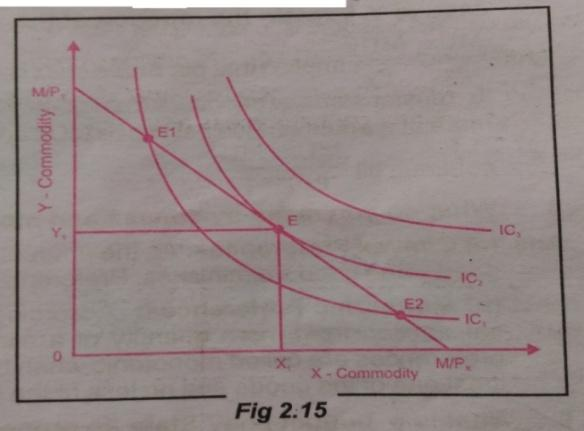The position of consumer’s equilibrium at point E, the budget line is tangent to the highest attainable indifference curve 1C2. Therefore, bundle E is the consumer’s optimum bundle. If the consumer buys more units of X beyond the equilibrium level,will become less thanand he will start losing. So he will not go beyond the equilibrium point. Similarly the consumer will not stop before the equilibrium level becausewill become greater than. It means that the consumer is willing to sacrifice more than he has to sacrifice actually to obtain one extra unit of X. So MRS must be equal to the ratio of prices of the two goods to obtain the position of equilibrium.
Q.9. Distinguish between normal goods and inferior goods.
 Normal Goods Inferior goods 1. These are the goods the demand for which increases as income of the buyer rises. 1. These are the goods the demand for which decreases as income of the buyer. 2. Diagram 2. Diagram 3.There is positive relationship between Income & demand for the good. 3. There is negative relationship between Income and demand for the good. 4. Example – Milk 4. Example – Coarse grain, Coarse cloth.

Q.10. State and explain the law of demand. 2012, 2014, 2016
Ans: “Other things remain constant” when the price of a commodity increases then its demand falls and when the price of the commodity decreases, then the demand increases for the commodity. That means, there is an inverse relationship between price of the commodity and its quantity. Other things constant means that consumer’s income, choice and preference of the consumer’s does not change. The law of demand is explained with the help of an individual demand schedule.
Individual Demand Schedule
 Price of orange (Per unit) Rs. Quantity of orange (Per unit) 2 4 6 8 10 8 6 4
From the above schedule it is shown that when the price of orange increases then the demand for orange decreases and it increases when its price decreases. This schedule is explained below with a diagram.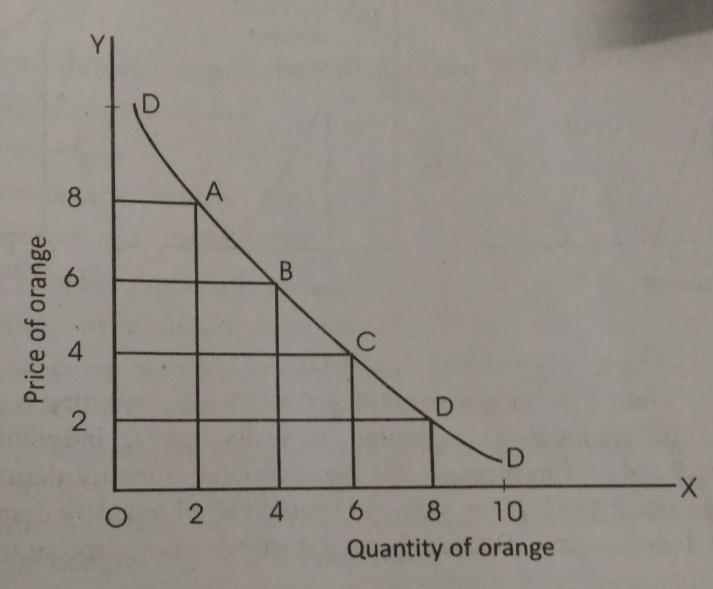In the diagram, OX-axis represents quantity of orange and OY-axis represents price of orange. When the price of orange is Rs. 8 then, the consumer will willing to purchase only 4 unit of oranges. But when the price of oranges falls to Rs. 2 then the demand of the consumer for oranges will go up to 10. If various points like A, B, C and D join together then the consumer gets a downward slopping curve which is the demand curve for the consumer. This curve is negatively sloped as there is an inverse relationship between price and quantity.
11. Why demand curve slopes downward?
Ans: The demand curve slopes downward from left to right. It is due to various reasons. Following are the sole reason given below:
1. It is for law of diminishing marginal utility; most of the buyers in the market follows according to this law. As the buyer purchases more of a commodity, he derives lesser amount of marginal utility from his consuming units. Therefore, he will purchase more of the commodity only if price falls.
2. If the price of the commodity falls, then consumer’s real income increases. So the consumer purchases a lot of commodity with his same money income.
3. Falling the price of a commodity created a new demand for that commodity or new customers for that commodity.
4. When the price of a commodity falls then consumer utilizes the commodity for several purposes. For the above mentioned reasons the demand curve slopes downward.
Q.12. Explain the factors that affect market demand for a commodity. 2016
Ans. Market demand for a commodity is affected by the following factors:
1. Price of the Commodity: When price of the commodity increases in the market, its quantity demanded decreases and vice versa.
2. Income of the Consumer: Market demand for a commodity is directly related to income of the consumer. Increase in income of consumer causes increase in market demand for the commodity.
3. Prices of Related Goods: In case of substitute goods, demand for a commodity falls with fall in price of the substitute commodity. In case of complementary goods, market demand for the commodity rises with a fall in the price of complementary commodity.
4. Tastes and Preferences: If consumer’s tastes and preferences change, quantity demanded of the commodity will also change.
5. Income Distribution: If income distribution is even, market demand for the commodity will be more, than otherwise.
6. Size of Population: Higher population implies greater market demand for goods and services and vice versa.
Q.13. Show the shifts in demand curve and explain the situation under which it changes. 2015
Ans: The demand curve will shifted if the consumer’s quantity demands of a commodity changes. Sometimes, the demand curve will change when the price of a commodity remains constant. There are various reason for the change in demand. Following are the most important ones:
1. Change in consumer’s income.
2. Change in the consumer’s tastes and habits.
3. Change in the relative preference of the consumer.
4. Change in the price of related commodities.
5. Expectation of decline in price in future etc.
In the below diagram, it is shown that at the same market price the demand curve is shifted fromtoor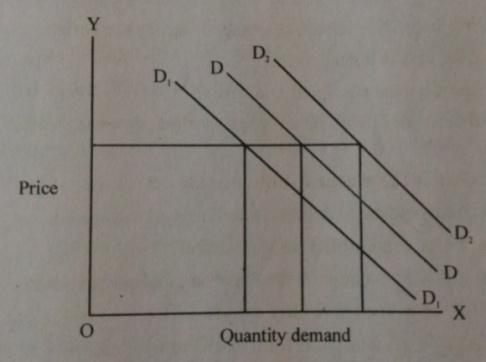Q.14. Prove that at consumer’s optimum print on indifference curve the marginal rate of substitution is equal to the ratio of the prices.
Ans: The budget like is included for determining optimum choice of the consumer and the point on the indifference curve. The point on the budget line which touches the indifference curve would be the optimum choice of the consumer. The following diagram shown the optimum choice of the consumer, where OX-axis represents X commodity and OY-axis represents Y commodity. At point E, consumer gets equilibrium. The budget line BB touches the indifference curve IC at point E. So, E is said to be the optional choice of the consumer at point E,. That means, Marginal rate of substitution is equal to the price ratio. At this point E, the slope of both the budget line and indifference curve is equal. Then assuming that the consumer spends his whole income on the two commodities X and Y and gets equal satisfaction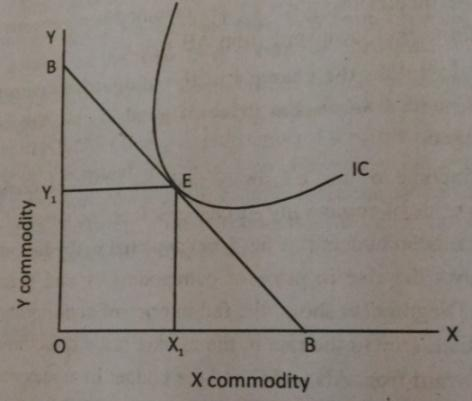Q.15. What are the different types of Price Elasticity of Demand?
Ans: There are various types of price elasticity of demand. These are given below:1. Inelastic Demand (or Relative Inelastic Demand): Demand is said to be relatively inelastic when the percentage change in demand is less than the percentage change in price. This means that the quantity demanded responds to a lesser extent than the given change in price. Necessary goods e.g. sugar, wheat, salt etc. are seen to have relatively inelastic demand. The value of elasticity of demand is less than one for a relatively inelastic demand.
2. Relatively Elastic Demand: Demand is said to be relatively elastic when the percentage change in demand is more than percentage change in price. This means that the quantity demanded responds to a greater extent than the given change in price.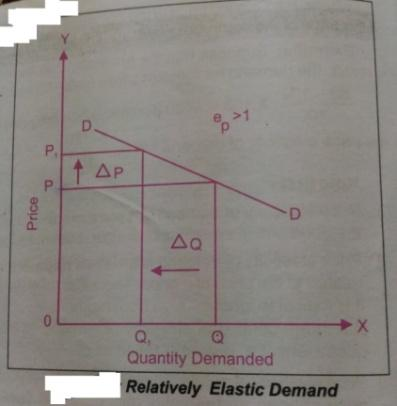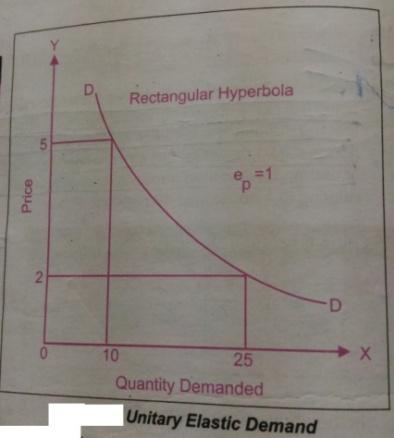1. Unitary Elastic Demand: When the percentage change in demand is equal to the percentage change in price of the commodity then demand is said to have unitary elasticity. The expenditure made by the consumer is always constant under unitary elasticity.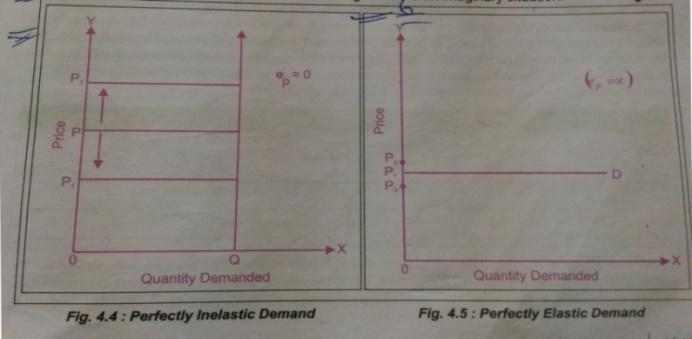1. Perfectly Inelastic Demand: Demand is perfectly inelastic when it does not change irrespective or of the changes in price.
2. Perfectly Elastic Demand: When the demand for a commodity reduces to zero with an infinitely small increase in price and increase to infinite with a very small reduction in price, then demand is said to be perfectly elastic.

Q.16. What are the different methods of measuring price elasticity of demand?
Ans: There are three methods of measuring price elasticity of demand:
1. Percentage method.
2. Total expenditure method.
3. Geometric method.
Percentage method/Proportionate method: This is the most popular method of measuring price elasticity of demand. Under this method, elasticity of demand is measured by the ratio of the proportionate (percentage) change is quantity demanded to proportionate (percentage) change in price. It is worked out as under:-Here,Price Elasticity of demand.Initial demand.Initial Price.New demand.New Price.Change in demand.Change in price.
Total Expenditure method: It measures price elasticity of demand on the basis of change in total expenditure, incurred on the commodity by a household as a result of change in its price. There are three conditions:
1. It TE on the commodity change inversely with the price change, the demand is relatively elastic (ed > 1)
2. If the total expenditure on the commodity remains the same as before and after change in price, then demand is said to be unitary (Ed = 1)
3. If the TE on the commodity increases with an increase in its price and decreases with a decrease in the price the demand is relatively inelastic (Ed < 1).
Geometric Method (2013): Geometric method was suggested by Prof. Marshall and is used to measure the elasticity at a point on the demand curve. When there are infinitely small changes in price and demand, then the ‘Geometric Method’ is used. This method is also known as ‘Graphic Method’ or ‘Point Method’ or ‘Arc Method’. Elasticity of demand (Ed) is different at different points on the same straight line demand curve. In order to measure Ed at any particular point, lower portion of the curve from that point is divided by the upper portion of the curve from the same point.
Elasticity of Demand (Ed) = Lower segment of demand curve (LS) / Upper segment of demand curve (US)
As seen in Fig. 4.1, elasticity at a particular point ‘N’ is calculated as NQ/NP.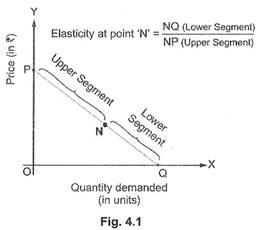Q.17. What is Price elasticity of demand? Explain the factor affecting price elasticity of demand. 2014, 2016
Ans: Ans. Price elasticity of demand as the ratio of the percentage change in quantity demanded to a percentage change in price. The elasticity of demand is affected by the following factors:
1. Nature of Commodity: Goods may be necessaries, luxuries and comforts. Demand for necessaries (like salt) is highly inelastic; demand for luxuries (like ACs) is highly elastic; and demand for comforts (like air coolers) is moderately elastic.
2. Availability of Substitutes: Commodities which have substitutes have elastic demand, like tea and coffee. Commodities having no substitutes like liquor and cigarettes, etc. have inelastic demand.
3. Alternative Uses of a Commodity: If a commodity is used for different purposes, it has elastic demand. Example: Electricity and coal.
4. Price Level: Higher the level of price, higher is the elasticity of demand for a commodity.
5. Time Period: Elasticity of demand is high over a long period (compared to a short period), because during a short period of time, consumption habits tend to be stable.
Q.18. How is the price elasticity of demand for a commodity affected by the nature of the commodity? Explain. 2013
Ans: Larger the number of substitutes of a commodity, greater is the elasticity of demand, as in a situation of monopolistic competition. This is because the consumer gets a choice of shifting from one commodity to the other when price of one commodity changes in relation to price of other. On the other hand, if a commodity has no close substitute as under monopoly, elasticity of demand will be low. Because, in such a situation the consumer has little choice of shifting from one commodity to the other when price changes.
Q.19. What are the causes of Increase in Demand?
Ans: An increase in demand for a commodity may be caused by any of the following factors:
1. Increase in the price of a substitute good.
2. A fall in the price of a complementary good.
3. Increase in the income level of consumers/households.
4. Change in tastes, preference, habits, climate, etc. in favour of a commodity.
Q.20. What factors cause a decrease in demand of a commodity?
Ans: A decrease in demand for a commodity may be caused by any of the following factors:
1. A fall in the price of a substitute good.
2. A rise in the price of a complementary good.
3. A fall in the income of the consumers/buyers.
4. Unfavourable changes in tastes, preferences, habits, climate etc.
21. Prove that at consumer’s optimum print on indifference curve the marginal rate of substitution is equal to the ratio of the prices. 2013, 2015
Ans. The budget like is included for determining optimum choice of the consumer and the point on the indifference curve. The point on the budget line which touches the indifference curve would be the optimum choice of the consumer. The following diagram shown the optimum choice of the consumer, where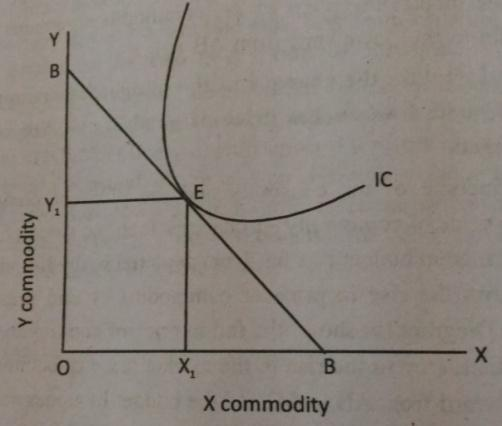OX-axis represents X commodity and OY-axis represents Y commodity. At point E, consumer gets equilibrium. The budget line BB touches the indifference curve IC at point E. So, E is said to be the optional choice of the consumer at point E,. That means, Marginal rate of substitution is equal to the price ratio. At this point E, the slope of both the budget line and indifference curve is equal. Then assuming that the consumer spends his whole income on the two commodities X and Y and gets equal satisfaction
Q.22. How market demand curve is derived from the individual demand curve? 2015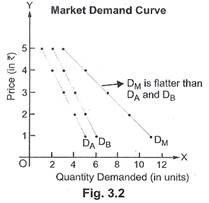Ans: Market Demand Curve: Market demand curve refers to a graphical representation of market demand schedule. It is obtained by horizontal summation of individual demand curves. The points shown in Table 3.2 are graphically represented in Fig. 3.2. DA and DB are the individual demand curves. Market demand curve (DM) is obtained by horizontal summation of the individual demand curves (DA and DB).

Market demand curve ‘DM‘ also slope downwards due to inverse relationship between price and quantity demanded.